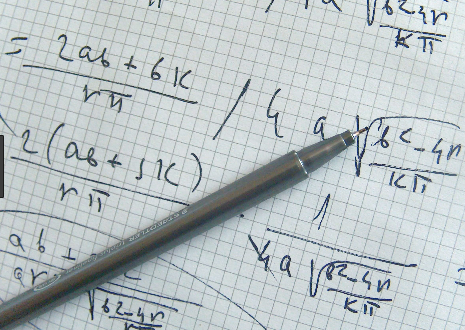# Specialist Maths

## SPECIALISTSpecialist Mathematics provides opportunities to develop rigorous mathematical arguments and proofs, and to use mathematical models extensively. Specialist Mathematics also extends understanding and knowledge of probability and statistics and introduces the topics of vectors, complex numbers and matrices.### Course Patterns

Students can form a minor, major, major-minor or double major in Specialist Mathematics by studying units from Specialist Methods in conjunction with this course.

### Unit 1

Topic 1: Combinatorics

Topic 2: Vectors in the Plane

Topic 3: Geometry

Combinatorics provides techniques that are

useful in many areas of mathematics, including probability and algebra. Vectors in the plane provides new perspectives on working with two-dimensional space, and serves as an introduction to techniques which can be ex-tended to three-dimensional space in Unit 3. Geometry extends studies in Euclidean Geometry, which is of benefit in the study of topics such as vectors and complex numbers.

### Unit 2

Topic 1: Trigonometry

Topic 2: Matrices

Topic 3: Real and Complex Numbers

Matrices provides new perspectives for working with two-dimensional space, Real and complex numbers provides a continuation of the study of numbers. Trigonometry contains techniques that are used in other topics in both this unit and Units 3 and 4. All of these topics develop students’ ability to construct mathematical arguments. The principle of mathematical induction is introduced.

### Unit 3

Topic 1: Complex Numbers

Topic 2: Functions & Sketching Graphs

Topic 3: Vectors in Three Dimensions

The Cartesian form of complex numbers is extended to the polar form. The study of functions and techniques of calculus is ex-tended and used in the sketching of graphs and problems involving integration. Vectors, is extended to three-dimensional vectors, vector equations and vector calculus, building on students’ knowledge of calculus. Cartesian and vector equations, and equations of planes, enable students to solve problems involving motion in three-dimensional space.

### Unit 4

Topic 1: Integrations & Applications

Topic 2: Rates of Change & Differential Equations

Topic 3: Statistical Inference

Differentiation and integration of functions is continued, and the techniques are applied to simple differential equations in biology and kinematics. These topics demonstrate the applicability of the mathematics in this course. Students’ experience in statistics is drawn together in the study of the distribution of sample means.